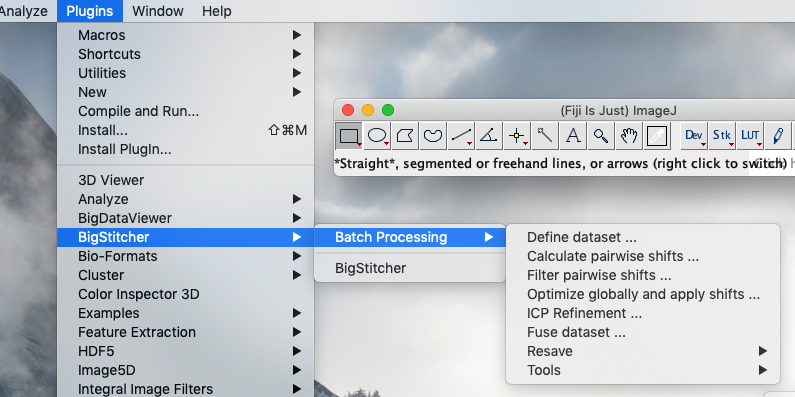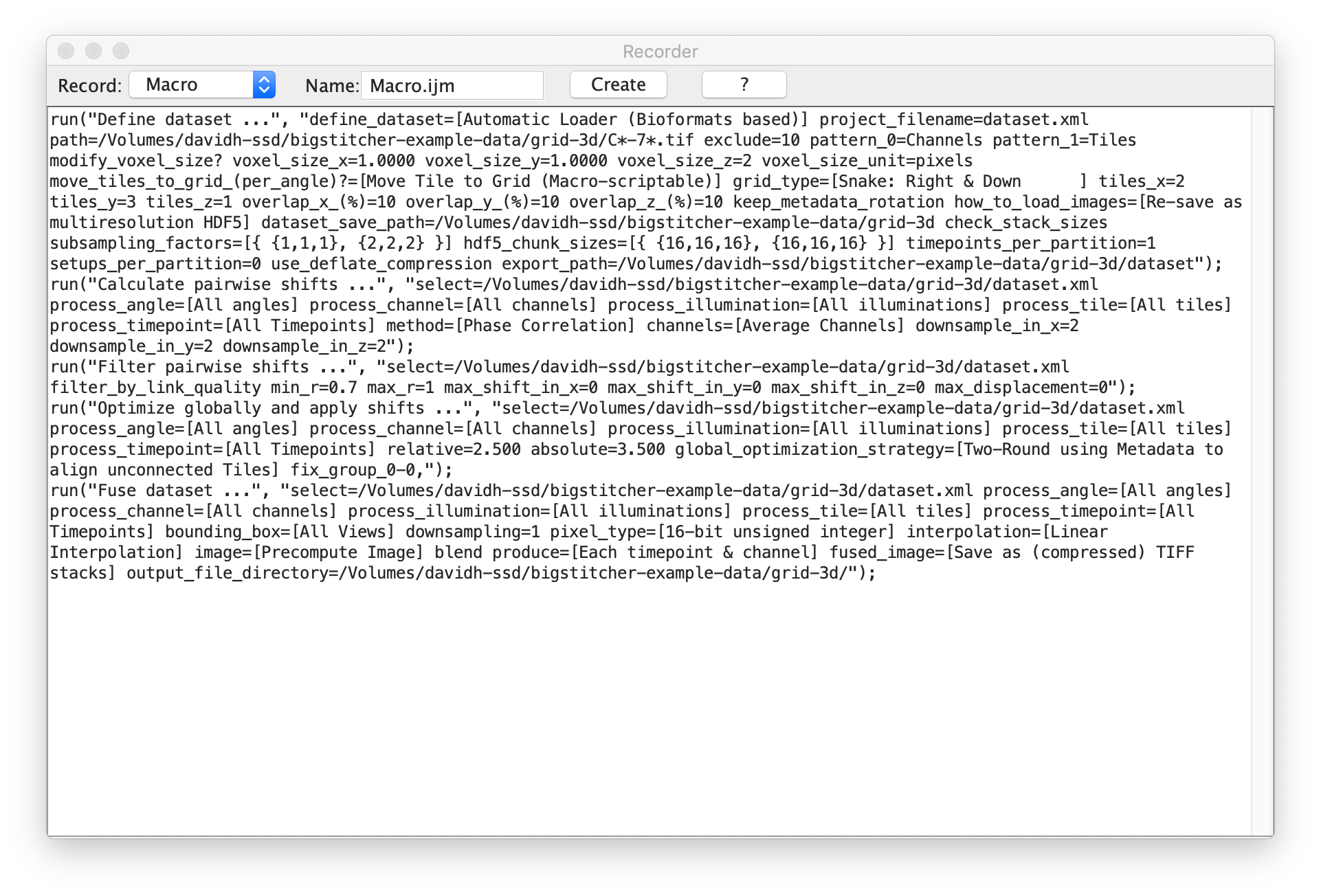The content of this page has not been vetted since shifting away from MediaWiki. If you’d like to help, check out the how to help guide!

## Overview

In addition to the main application available in the Fiji menu via PluginsBigStitcherBigStitcher, we offer macro-recordable versions of most processing steps under the PluginsBigStitcherBatch Processing menu.The operation of the Batch versions of the processing steps is essentially the same as in the main application, though with a more rudimentary UI that just asks for parameters before performing the processing step.

Macro-scriptable versions of the Multiview Reconstruction steps can be found in the Fiji menu under: PluginsMultiview ReconstructionBatch Processing

## Example: Recording processing steps

Clicking PluginsMacrosRecord… in the Fiji will bring up the macro recorder that compiles most actions performed in Fiji as a executable script.

In the following example, we performed the following macro-recordable steps of BigStitcher:

• Import a dataset (available here), arange the tiles into a regular grid and re-save the data as HDF5.
• Perform pairwise shift calculation via Phase correlation, filter the links by thresholding on the correlation coefficient and globally optimize the alignment.
• Fuse the Tiles and save the results as TIFFClicking Generate in the macro recorder will pop up the Fiji script editor with a new script containing the recorded commands. You can save or Run the created script.

## Example: Modifying and Calling the macro

Calling the macro generated after clicking Generate will just perform the same steps on the same dataset again. By providing some parameters to the script as an argument string and substitution them in the recorded commands, a script for headless, batch operation of BigStitcher can be generated.

In the example below, the user can pass a parameter string of the form

` /path/to/data num_tiles_x num_tiles_x`

to process another dataset with a different number of tiles headlessly:

``````// read dataset path, number of tiles as commandline arguments
args = getArgument()
args = split(args, " ");

basePath = args;
if (!endsWith(basePath, File.separator))
{
basePath = basePath + File.separator;
}
tilesX = args;
tilesY = args;

// define dataset
run("Define dataset ...",
" project_filename=dataset.xml path=" + basePath + "C*-7*.tif exclude=10" +
" pattern_0=Channels pattern_1=Tiles modify_voxel_size? voxel_size_x=1.0000" +
" voxel_size_y=1.0000 voxel_size_z=2 voxel_size_unit=pixels " +
"move_tiles_to_grid_(per_angle)?=[Move Tile to Grid (Macro-scriptable)] grid_type=[Snake: Right & Down      ]" +
" tiles_x="+tilesX+" tiles_y="+tilesY+" tiles_z=1 overlap_x_(%)=10 overlap_y_(%)=10 overlap_z_(%)=10" +
"dataset_save_path=/Volumes/davidh-ssd/bigstitcher-example-data/grid-3d check_stack_sizes " +
"subsampling_factors=[{ {1,1,1}, {2,2,2} }] hdf5_chunk_sizes=[{ {16,16,16}, {16,16,16} }] " +
"timepoints_per_partition=1 setups_per_partition=0 use_deflate_compression " +
"export_path=" + basePath + "dataset");

// calculate pairwise shifts
run("Calculate pairwise shifts ...",
"select="+basePath+"dataset.xml process_angle=[All angles] process_channel=[All channels]" +
" process_illumination=[All illuminations] process_tile=[All tiles] process_timepoint=[All Timepoints]" +
" method=[Phase Correlation] channels=[Average Channels] downsample_in_x=2 downsample_in_y=2 downsample_in_z=2");

// filter shifts with 0.7 corr. threshold
run("Filter pairwise shifts ...",
"select="+basePath+"dataset.xml filter_by_link_quality min_r=0.7 max_r=1 " +
"max_shift_in_x=0 max_shift_in_y=0 max_shift_in_z=0 max_displacement=0");

// do global optimization
run("Optimize globally and apply shifts ...",
"select="+basePath+"dataset.xml process_angle=[All angles] process_channel=[All channels] " +
"process_illumination=[All illuminations] process_tile=[All tiles] process_timepoint=[All Timepoints]" +
" relative=2.500 absolute=3.500 global_optimization_strategy=" +
"[Two-Round using Metadata to align unconnected Tiles] fix_group_0-0,");

// fuse dataset, save as TIFF
run("Fuse dataset ...",
"select="+basePath+"dataset.xml process_angle=[All angles] process_channel=[All channels] " +
"process_illumination=[All illuminations] process_tile=[All tiles] process_timepoint=[All Timepoints]" +
" bounding_box=[All Views] downsampling=1 pixel_type=[16-bit unsigned integer] interpolation=[Linear Interpolation]" +
" image=[Precompute Image] blend produce=[Each timepoint & channel] fused_image=[Save as (compressed) TIFF stacks] " +
"output_file_directory=" + basePath);

// quit after we are finished
eval("script", "System.exit(0);");
``````

After saving the macro, it can be run from any Terminal by starting Fiji in Headless mode and passing the macro as well as a parameter string.

` /path/to/fiji/ImageJ-linux64 –headless –console -macro /path/to/macro/bigStitcherBatch.ijm “/path/to/data 2 3”`

Go back to the main page